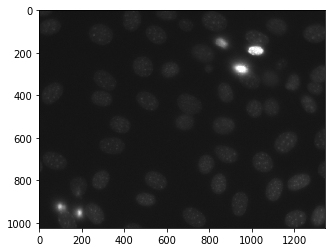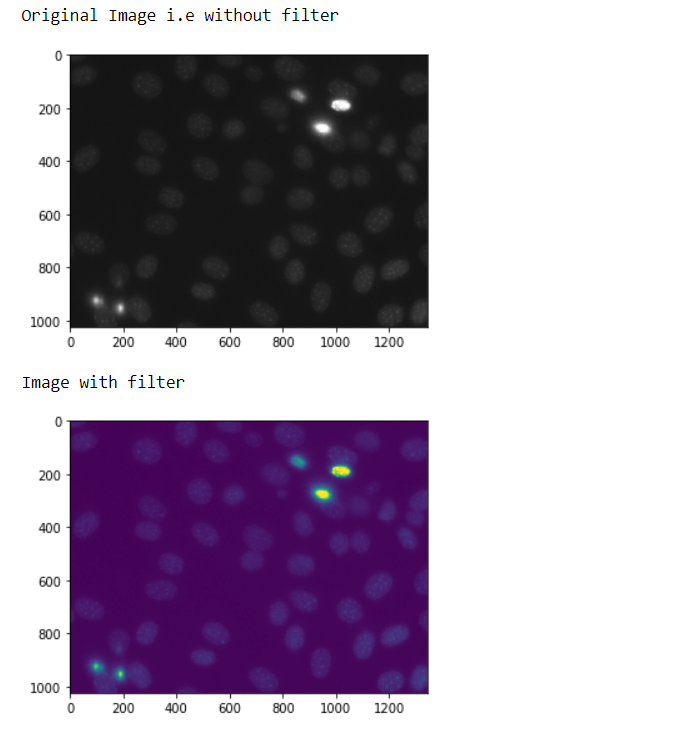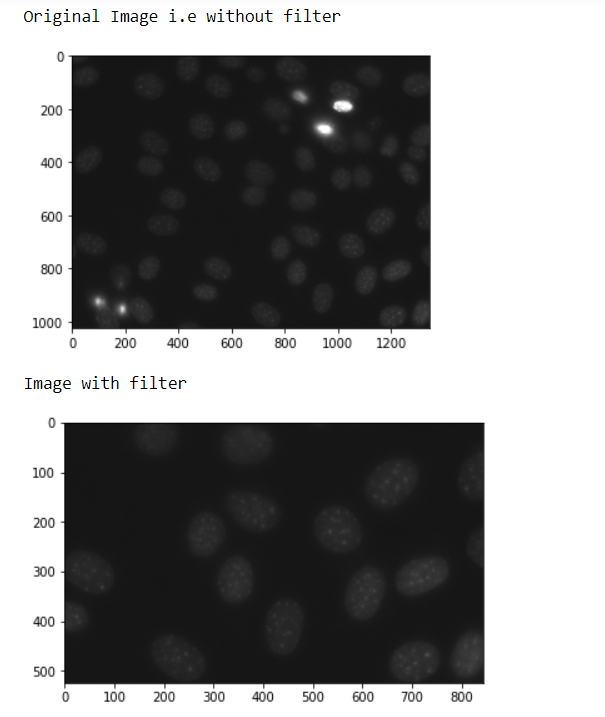Related Articles

# Mahotas – Filtering Region

• Last Updated : 21 Jun, 2020

In this article, we will see how we can filter region in mahotas. For this, we are going to use the fluorescent microscopy image from a nuclear segmentation benchmark. We can get the image with the help of command given below –

`mhotas.demos.nuclear_image()`

Below is the nuclear_imageIn order to filter this image we will take the image object which is numpy.ndarray and filter it with the help of indexing, below is the command to do this

`nuclear = nuclear[:, :, 0]`

Example 1 :

 `# importing required libraries``import` `mahotas as mh``import` `mahotas.demos``import` `numpy as np``from` `pylab ``import` `imshow, show`` ` `# getting nuclear image``nuclear ``=` `mh.demos.nuclear_image()`` ` `print``(``"Original Image i.e without filter"``)``# show the original image``imshow(nuclear)``show()`` ` `# filtering the image``nuclear ``=` `nuclear[:, :, ``0``]`` ` `print``(``"Image with filter"``)``# showing the image``imshow(nuclear)``show()`

Output :Example 2 :

 `# importing required libraries``import` `mahotas as mh``import` `mahotas.demos``import` `numpy as np``from` `pylab ``import` `imshow, show`` ` `# getting nuclear image``nuclear ``=` `mh.demos.nuclear_image()`` ` `print``(``"Original Image i.e without filter"``)``# show the original image``imshow(nuclear)``show()`` ` `# filtering the image``nuclear ``=` `nuclear[``500``:, ``500``:, :]`` ` `print``(``"Image with filter"``)``# showing the image``imshow(nuclear)``show()`

Output :Attention geek! Strengthen your foundations with the Python Programming Foundation Course and learn the basics.

To begin with, your interview preparations Enhance your Data Structures concepts with the Python DS Course. And to begin with your Machine Learning Journey, join the Machine Learning – Basic Level Course

My Personal Notes arrow_drop_up